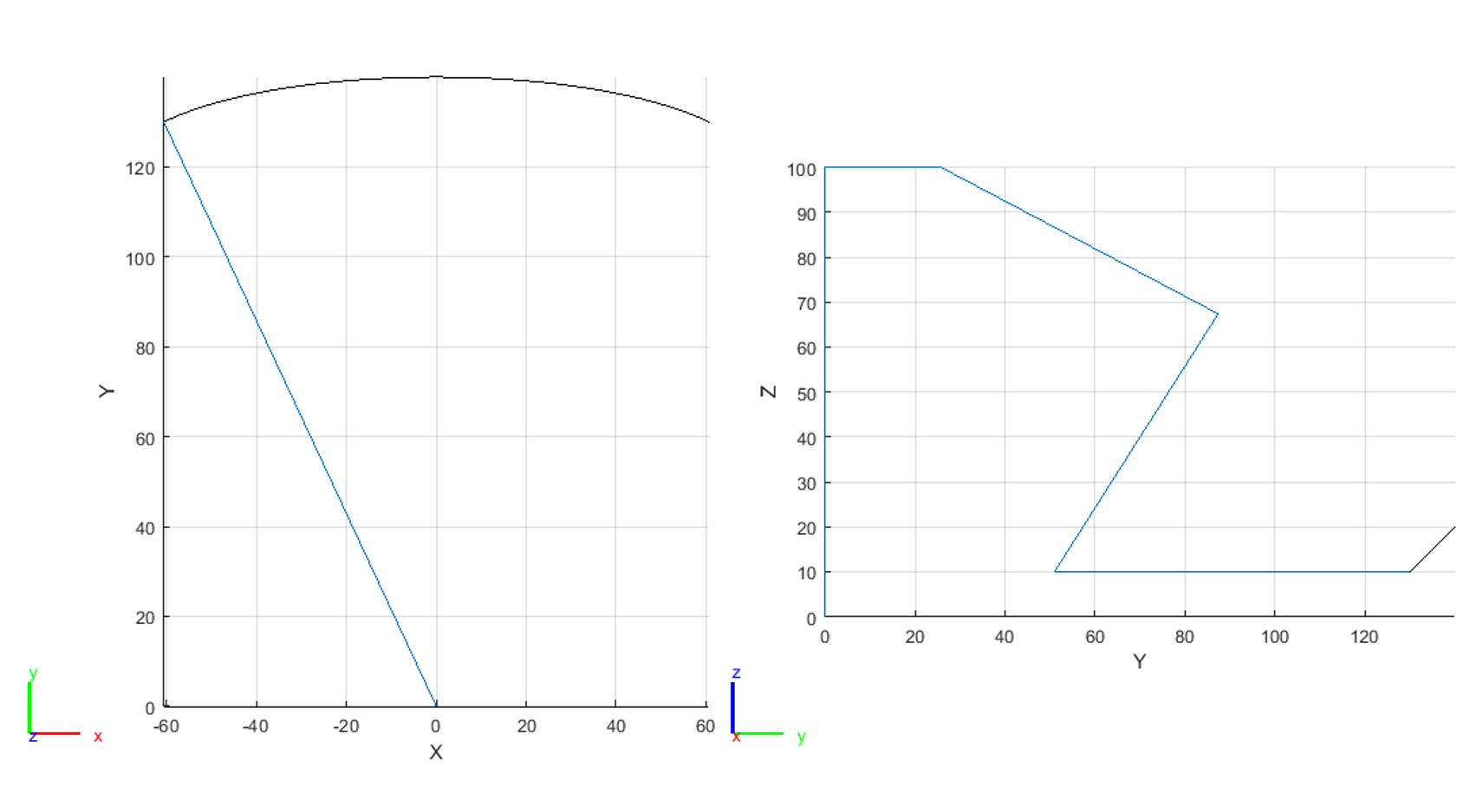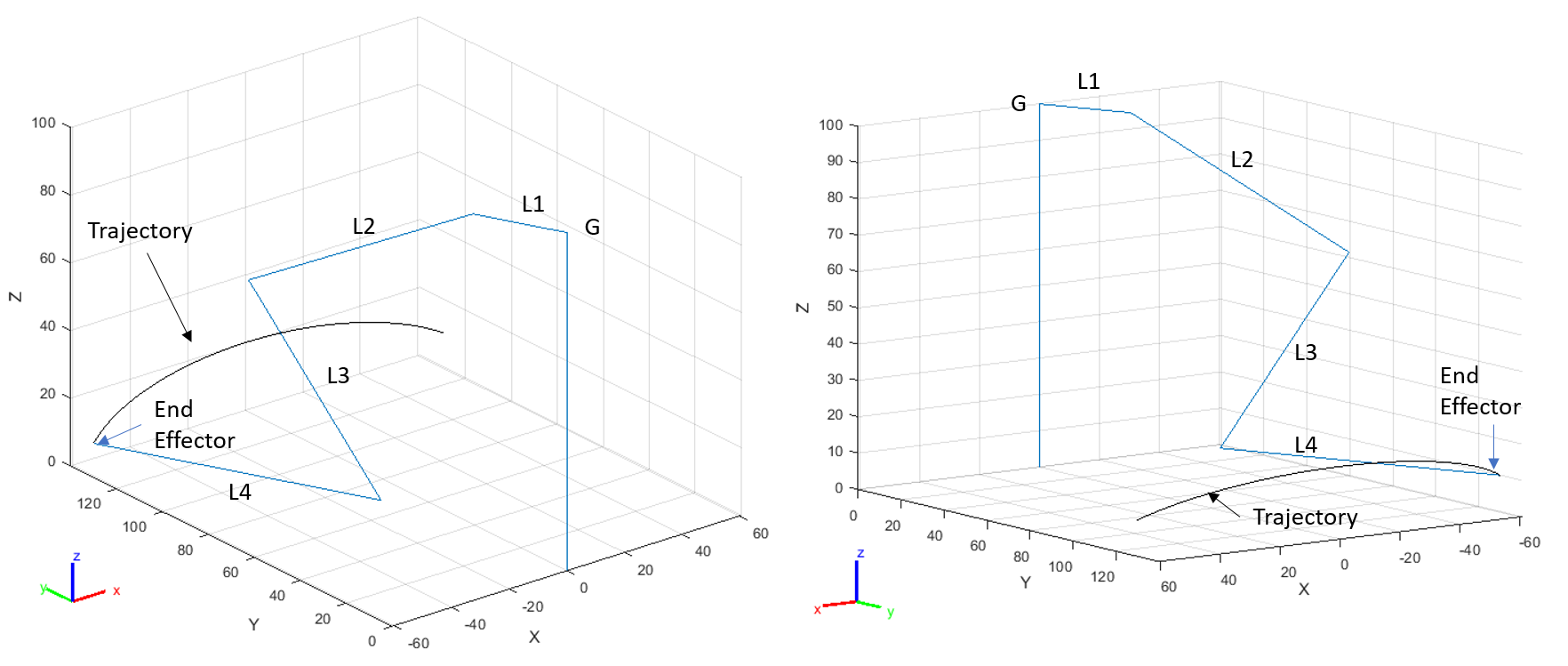The Dof Community was shut down in June 2023. This is a read-only archive.
If you have questions about Robotiq products please reach our support team.jgk5141

I am trying to apply inverse kinematics to robot leg that has 4 degrees of freedom. I do not know how to think about solving this problem, so any guidance would be greatly appreciated!

My first question is, is inverse kinematics the correct approach? To me it seems to be since I want to determine the angles for the servos based on the foot position and trajectory. If anyone has any recommendations with respect to this I would greatly appreciate it so that I know am headed down the right path. That being said, I am struggling to find solutions to problems of this sort. It seems as though 4 DoF is more challenging and is often not solved analytically. I would still like to solve this analytically, but I have moved on to try out numerical solutions.

In MATLAB, I have worked through several examples and I have tried to transition them to the case of this system. I have attached my code so far. Currently, I am struggling with applying joint constraints, getting the configuration that is more physical, and getting a useable solution from this approach. So my questions to this are:

1. Is this a valid approach to finding the solution to inverse kinematics of this system?
• As in, if I manage to get this to work, is the solution useable? I may be picturing this wrong, but I foresee getting a sequence of servo angles that will correspond to the trajectory. If I get these servo angles (and the joint constraints, lengths, etc. are all physically accurate) will I be able to give the leg the sequence? If this is even a valid approach, I have some MATLAB questions to follow.
2. Will this approach transition well to when there are more legs (i.e. the complete robot with 6 legs)?
3. How do joint constraints work in the robotics toolbox?
• It seems like rotation is right-handed with respect to the axis of rotation. In addition, when a positive angle is applied, it seems to rotate the rigidbody below the local coordinate system of the joint. However, there are joint constraints to the servos and when I try to apply these, the IK solver that I setup fails to follow the defined trajectory and will give errors saying that the joint angle is outside of the constraints set, even when I make sure that they are valid ranges. I have not been able to find useful documentation on this.
4. If I get the joint constraints set correctly, will the solution be in the correct configuration?
• Should I consider other constraints to apply?
• Is the inverseKinematics from MATLAB what I need or should I be trying generalizedInverseKinematics?
5. Currently, I defined some random arc for the trajectory. Should I consider something else for the walk of a hexapedal robot?

I am open to any suggestions since it is clear that I do not have any direction. Please let me know if more information is needed and I will edit the post. Physical dimensions of the robot are included in the code. The height of base is arbitrary currently. I have tried to comment the code heavily so that it is easy to understand. Lastly, here are 4 images showing the current results of the MATLAB simulation. blue lines are the body segments and the black ark is the trajectory.Code:

`% Robot_Advanced_Real.m`

``````% Copied from matlab and adapted for my scenario.
% Creates robot, uses IK to calculate positions, Creates animation

% Initialize MATLAB
clear all;
close all;
clc;
tic;

%%%%%%%%%%%%%%%%%%%%%%%%%%%%%%%%%%%%%%%%%%%%%%%%%%%%%%%%%%%%%%%%%%%%
%% Building Robot
%%%%%%%%%%%%%%%%%%%%%%%%%%%%%%%%%%%%%%%%%%%%%%%%%%%%%%%%%%%%%%%%%%%%

robot = rigidBodyTree('DataFormat','column','MaxNumBodies',5);

% Specify Arm Lengths of the Robot Arm. Units: [mm]
L1 = 28.45;
L2 = 75.41;
L3 = 70.02;
L4 = 87.14;

% Height of robot body off of Ground
G = 100;

% Add first body with joint. Set as robot base.
joint1 = rigidBodyJoint('joint1', 'revolute');
setFixedTransform(joint1,trvec2tform([0 0 G]));
joint1.JointAxis = [0 0 1];
joint1.HomePosition = pi/6;
% joint.PositionLimits = [0, pi];
body.Joint = joint1;

% Add second body with joint. Attach to First
joint2 = rigidBodyJoint('joint2','revolute');
setFixedTransform(joint2, trvec2tform([L1,0,0]));
joint2.JointAxis = [0 1 0];
joint2.HomePosition = pi/6;
% joint.PositionLimits = [0, pi];
body.Joint = joint2;

% Add third body with joint. Attach to second
joint3 = rigidBodyJoint('joint3','revolute');
setFixedTransform(joint3, trvec2tform([L2,0,0]));
joint3.JointAxis = [0 1 0];
joint3.HomePosition = pi/6;
% joint.PositionLimits = [0, pi];
body.Joint = joint3;

% Add fourth body with joint. Attach to third
joint4 = rigidBodyJoint('joint4','revolute');
setFixedTransform(joint4, trvec2tform([L3,0,0]));
joint4.JointAxis = [0 1 0];
joint4.HomePosition = pi/6;
% joint.PositionLimits = [0, pi];
body.Joint = joint4;

% Add End Effector with Fixed Joint. Attach to final body.
body = rigidBody('tool');
joint = rigidBodyJoint('fix1','fixed');
setFixedTransform(joint, trvec2tform([L4, 0, 0]));
body.Joint = joint;

% View Robot Input details to review setup
showdetails(robot);

%%%%%%%%%%%%%%%%%%%%%%%%%%%%%%%%%%%%%%%%%%%%%%%%%%%%%%%%%%%%%%%%%%%%
%% Define Trajectory
%%%%%%%%%%%%%%%%%%%%%%%%%%%%%%%%%%%%%%%%%%%%%%%%%%%%%%%%%%%%%%%%%%%%

% Define series of [x y z] for arc step.
% Define parameters of the arc.
xCenter = 0;
yCenter = 120;
zCenter = 0;

% Define the angle theta as going from 30 to 150 degrees in 100 steps.
theta = linspace(30, 150, 100);
% Define x and y using "Degrees" version of sin and cos.
x = (xRadius * cosd(theta) + xCenter)';
y = (yRadius * sind(theta) + yCenter)';
z = (zRadius * sind(theta) + zCenter)';

% Points around circle
% points = center + [x y z];
points = [x y z];
count  = length(x);

% Check
% plot3(points(:,1),points(:,2),points(:,3))
plot3(x,y,z)
hold on
plot3(points(:,1),points(:,2),points(:,3),'k')
xlabel('x')
ylabel('y')
zlabel('z')

%%%%%%%%%%%%%%%%%%%%%%%%%%%%%%%%%%%%%%%%%%%%%%%%%%%%%%%%%%%%%%%%%%%%
%% Inverse Kinematics
%%%%%%%%%%%%%%%%%%%%%%%%%%%%%%%%%%%%%%%%%%%%%%%%%%%%%%%%%%%%%%%%%%%%

% Use IK object to find a solution to the joint configurations that achieve
% end effector coordinates along ttrajectory

% Pre-allocate configuration solutions as a matrix, qs
q0 = homeConfiguration(robot);
ndof = length(q0);
qs = zeros(count, ndof);

% create the IK Solver.
ik = inverseKinematics('RigidBodyTree', robot);

% Use desired weights for solution (First three are orientation, last three are translation)
% Since it is a 4-DOF robot with only one revolute joint in Z we do not
% put a weight on Z rotation; otherwise it limits the solution space
% weights = [0, 0, 0, 1, 1, 0];
weights = [0.1, 0.1, 0, 1, 1, 1];
endEffector = 'tool';

% Loop through the trajectory and store the configurations. IK is used to
% give joint configurations.
qInitial = q0; % Use home configuration as the initial guess
for i = 1:count
% Solve for the configuration satisfying the desired end effector
% position
point = points(i,:);
qSol = ik(endEffector,trvec2tform(point),weights,qInitial);
% Store the configuration
qs(i,:) = qSol;
% Start from prior solution
qInitial = qSol;
end

%%%%%%%%%%%%%%%%%%%%%%%%%%%%%%%%%%%%%%%%%%%%%%%%%%%%%%%%%%%%%%%%%%%%
%% Animate Solution
%%%%%%%%%%%%%%%%%%%%%%%%%%%%%%%%%%%%%%%%%%%%%%%%%%%%%%%%%%%%%%%%%%%%

% Show first configuration. Show trajectory.
figure
show(robot,qs(1,:)');
ax = gca;
ax.Projection = 'orthographic';
hold on
plot3(points(:,1),points(:,2),points(:,3),'k')
view([-37.5 30])

% Set up animation. Show robot in each configuration on trajectory
framesPerSecond = 7;
r = rateControl(framesPerSecond);
for i = 1:count
show(robot,qs(i,:)','PreservePlot',false);
drawnow
waitfor(r);
end

toc``````